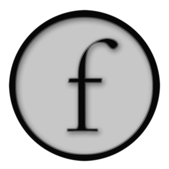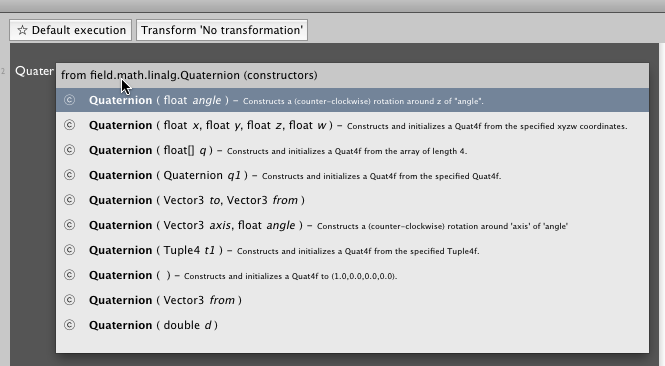Simple Linear Algebra

Somewhere on the path between computer science and art inevitably lies the town of linear algebra: Vectors, rotations, matrices and so on. Field comes with a number of well-populated classes to make manipulating these things slightly less tedious.

Here's a quick and incomplete set of examples to give you a flavor of `Vector2`, which is used to represent a point or a direction in 2d space:

``````v1 = Vector2() # a vector at 0,0
v2 = Vector2(1, -2.5) # a vector at 1, -2.5

#they act a lot like Python tuples
print v2, v2.x # prints 1, 1
print v2, v2.y # prints -2.5, -2.5

#which mean you can pass them to PLine methods using * notation
PLine().moveTo( *v2 ) # is the same as .moveTo(1, -2.5)

v3 = v1+v2 # vectors can be added
v4 = v2*2.0 # vectors can be scaled
v5 = v2*v1 # vectors can be element-wise multipled
v1 += Vector2(5,5) #vectors can be mutated in place
``````

`Vector2` also has all manner of useful methods:

``````v1.normalize() # makes the length 1
dot = v1.dot(v2) # dot product
angle = v1.angle(v2) # the angle between v1 and v2
v6 = v1.orthogonal() # a vector2 that's at right angles to this one
v1.projectOut(v2) # remove part of the vector that's pointing in the direction v2

v1.interpolate(v2, 0.25) # move 25% of the way towards v2
``````

By convention, any method that doesn't have anything better to return, returns the `Vector2` that it operated on. This means that you can chain things together:

``````print v1.normalize().scale(5).projectOut(v2)+v3
``````

Likewise, `Vector3`, `Vector4`, `Color4`, `VectorN` (an 'any dimension' Vector). See also Quaternion.

"See also?" Yes, Field contains its best source of documentation about this kind of thing: autocompletion is always the most authoritative way of getting info on these kinds of classes. Since it reads the source files that are ultimately compiled into your `Vector2`, it's never out of date and always complete. Also, don't miss right-click "open in Eclipse" and "search openendedgroup.com/field" options in the text editor.

For example, wondering how to get a `Quaternion`? press command-period after typing `Quater`:Finally, `Vector2,3,4,N` can be "sliced" as in Python lists:

``````v = Vector4(1,2,3,4)
print v[:] # prints [1,2,3,4]

v[:] = (4,3,2,1) # sets this vector to a new value
print v # prints [4,3,2,1]

print v[0:2] # prints [4,3]
``````

This is sometimes very useful for setting the values inside a `VectorX` very tersely.

This means that they can also be passed into functions for argument lists:

``````FLine().moveTo(*(a+b)*0.2)
``````

Is equivalent to (and faster than):

``````FLine().moveTo( ((a+b)*0.2).x, ((a+b)*0.2).y, ((a+b)*0.2).z)
``````

CFrame

CFrame is Field's coordinate frame factory function. Coordinate frames are useful for describing combinations of transformations in 3d spaces — compositions of rotations, translations and scales. Two things that you need to know about `CFrame`s — firstly, is that the function you get them from tries to be maximally helpful; secondly, you can multiply them together to create new ones. First the function:

``````CFrame(r=Quaternion(), s=Vector3(1,1,1), t=Vector3(0,0,0), center=None)
``````

CFrame is very obliging when it comes to the types of the things that you pass in. Here's a rotation of Pi/2 around the z axis:

``````r = CFrame(r=Math.PI/2)
``````

A uniform scale of 0.5 around the point `Vector3(100, 20, 40)`

``````r = CFrame(s=0.5, center=Vector3(100,20,40))
``````

CFrame will take anything for 'r' that we have a constructor for in `Quaternion`. Here's a rotation that takes the y-axis over to the x-axis:

``````r = CFrame(r=[Vector3(1,0,0),Vector3(0,1,0)])
print r * Vector3(0,1,0) # -- prints something close to [1,0,0]
``````

Secondly, these things can be multiplied together:

``````r1 = CFrame(r=[Vector3(1,0,0),Vector3(0,1,0)])
r2 = CFrame(s=0.5, center=Vector3(100,0,0))
r3 = CFrame(t=Vector3(0,50,0))

total = r3*r2*r1

print total * Vector3(0,1,0) # -- prints [50,50,0]
``````

Mentally you should think of multiplication as producing a machine. The `Vector3` goes in the right hand side and comes out the left had side, passing through each of the operations in turn.